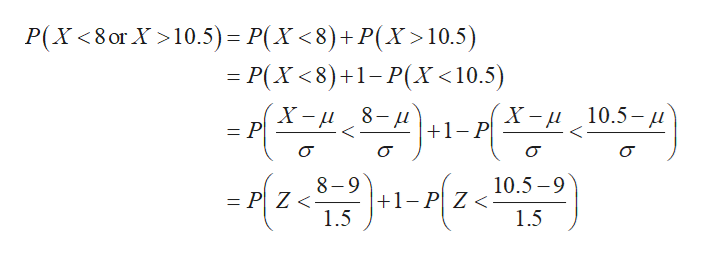# Normal Distribution Problem: A certain component for the newly developed electronic diesel engine is considered to be defective if its diameter is less than 8 mm or greater than 10.5 mm. The distributions of the diameters of these parts is known to be normal with a mean of 9.0 mm and a standard deviation of 1.5 mm. If a component is randomly selected, what is the probability that it will be defective?Q: Do I add the Right and Left tails and that's my total probability of defectiveness? Or, Do I take the Normal.Dist (from Excel) to the left of 10.5 minus the N.D left of 8 for a total of .588852 probability that it will be defective (that's a lot)?Please help! And, thank you in advance!

Question
112 views

Normal Distribution Problem: A certain component for the newly developed electronic diesel engine is considered to be defective if its diameter is less than 8 mm or greater than 10.5 mm. The distributions of the diameters of these parts is known to be normal with a mean of 9.0 mm and a standard deviation of 1.5 mm. If a component is randomly selected, what is the probability that it will be defective?

Q: Do I add the Right and Left tails and that's my total probability of defectiveness? Or, Do I take the Normal.Dist (from Excel) to the left of 10.5 minus the N.D left of 8 for a total of .588852 probability that it will be defective (that's a lot)?

check_circle

star
star
star
star
star
1 Rating
Step 1

Given information:

Mean = 9.0 mm

Standard deviation = 1.5 mm

Step 2

Let x is random variable.

Now, the probability that it will be defective is calculated as follows:help_outlineImage TranscriptioncloseP(X<8or X10.5) P(X<8)+P(X>10.5) = P(X <8)+1-P(X<10.5) X-10.5-u X -и 8-u 1 P = P 8-9 +1-P Z 1.5 10.5-9 = P Z < 1.5 fullscreen
Step 3

Simplify further a...

### Want to see the full answer?

See Solution

#### Want to see this answer and more?

Solutions are written by subject experts who are available 24/7. Questions are typically answered within 1 hour.*

See Solution
*Response times may vary by subject and question.
Tagged in

### Statistics